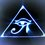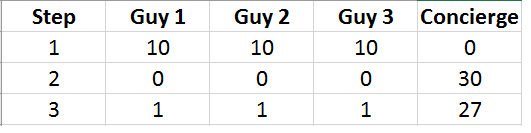# The Missing Dollar

Three guys walk into a hotel and they are tight for cash so they decide to split one room. The concierge at the counter checks them in and when it comes time to pay he asks them for $30. Since there are three of them they decide to split the bill evenly and they each pay$10. Later that night the concierge realizes he has made a terrible mistake. He charged them for a luxury suite when they only got a regular suite. The price of the regular suite was only $25. He realizes that if he gives them back a$5 bill they will not be able to split it evenly. His solution to this problem is to give them each back $1 in the morning (giving back a total of 3$) and then he pockets the other $2 as a tip. Here is where the problem arises. If they each originally paid 10$ but they each got 1$back, then it is as if they have each now paid 9$. If they each paid 9$and there are three of them, and$9 times 3 is equal to $27. Then it would seem they paid$27 for the room. Of course the concierge also took a $2 tip. So$27 plus $2 gives a grand total of$29. But originally they had paid \$30. Where is the missing dollar.

Can you discover where the error occurs?Note by Brody Acquilano
1 year, 11 months ago

This discussion board is a place to discuss our Daily Challenges and the math and science related to those challenges. Explanations are more than just a solution — they should explain the steps and thinking strategies that you used to obtain the solution. Comments should further the discussion of math and science.

When posting on Brilliant:

• Use the emojis to react to an explanation, whether you're congratulating a job well done , or just really confused .
• Ask specific questions about the challenge or the steps in somebody's explanation. Well-posed questions can add a lot to the discussion, but posting "I don't understand!" doesn't help anyone.
• Try to contribute something new to the discussion, whether it is an extension, generalization or other idea related to the challenge.

MarkdownAppears as
*italics* or _italics_ italics
**bold** or __bold__ bold
- bulleted- list
• bulleted
• list
1. numbered2. list
1. numbered
2. list
Note: you must add a full line of space before and after lists for them to show up correctly
paragraph 1paragraph 2

paragraph 1

paragraph 2

[example link](https://brilliant.org)example link
> This is a quote
This is a quote
    # I indented these lines
# 4 spaces, and now they show
# up as a code block.

print "hello world"
# I indented these lines
# 4 spaces, and now they show
# up as a code block.

print "hello world"
MathAppears as
Remember to wrap math in $$ ... $$ or $ ... $ to ensure proper formatting.
2 \times 3 $2 \times 3$
2^{34} $2^{34}$
a_{i-1} $a_{i-1}$
\frac{2}{3} $\frac{2}{3}$
\sqrt{2} $\sqrt{2}$
\sum_{i=1}^3 $\sum_{i=1}^3$
\sin \theta $\sin \theta$
\boxed{123} $\boxed{123}$

Sort by:

The 2 dollar tip is on top of the 25 dollar actual price, so the concierge ends up with 27 dollars, and each guy ends up with 1 dollar, for a total of 30 dollars. The steps are shown below.- 1 year, 11 months ago

As Steven chase pointed out the proper way to approach this problem is:

$10+10+10= 30$ $10+10+10-3= 30- 3$ $(10-1)+(10-1)+(10-1)= 30-3$ $9+9+9=27$

The error arises as follows. Notice in step three we forget to subtract the three dollars from the right hand side of the equation In step five we add two dollars back to the left hand side, but again we don't do anything to the right hand side. If we subtracted three dollars from the left hand side and then add two dollars to the left hand side then the left hand side is off by one.

$10+10+10= 25 +5$ $10+10+10= 25 + 3+ 2$ $10+10+10-3=25+2+3$ $(10-1) + (10-1)+ (10-1) = 25+2+3$ $9 + 9+ 9 +2 = 25+2+3$ $29 = 30$

- 1 year, 11 months ago